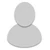## FANDOM

857 Pages

• For prime numbers which are over 5,000. There is no way to represent them in roman numerals. What will we do when we encounter this issue? The largest roman number is M which dictates 1,000. Would the roman numberal  for 5,003 be like this?

Would it be like this-- MMMMMIII

I also saw some sources that added a line above one of the already created numbers. The lines meant that the aforementioned number was multiplied by 1,000

_

for example =V III

Which method would we choose? If we choose the second way, how will we implement the line?

If this wiki ever goes above 1,000,000 we ought to add 2 lines and 1,000,000,000, 3 and so one.

How shall we address this issue? Should we just ditch roman numberals coloum for primes 5,000 and above?

• Interesting problem that I also don't have a solution to. I think removing roman numerals above number 5000 will be the best way to deal with.

But still I found a way to add overlines like this, so why not:

• 5407 ==> VCDVII
• 157897 ==> CLVMMDCCCXCVII

This is also very useful for representing rational numbers that have repeating digits such as this:

• 1/31 = 0.032258064516129

I added the template Template:Overline for this.

•